# 1.7. 高斯过程

## 1.7.1. 高斯过程回归（GPR）

GaussianProcessRegressor 类实现了回归情况下的高斯过程(GP)模型。 为此，需要实现指定GP的先验。当参数 normalize_y=False 时，先验的均值 通常假定为常数或者零; 当 normalize_y=True 时，先验均值通常为训练数 据的均值。而先验的方差通过传递 内核(kernel) 对象来指定。通过 最大化基于传递 optimizer 的对数边缘似然估计(LML)，内核的超参可以在 GaussianProcessRegressor 类执行拟合过程中被优化。由于 LML 可能会存在多个 局部最优解，因此优化过程可以通过指定 n_restarts_optimizer 参数进行 多次重复。通过设置内核的超参初始值来进行第一次优化的运行。后续的运行 过程中超参值都是从合理范围值中随机选取的。如果需要保持初始化超参值， 那么需要把优化器设置为 None

• 允许预测，无需事先拟合（基于GP先验）
• 提供了一种额外的方法 sample_y(X) , 其评估 在给定输入处从 GPR （先验或后验）绘制的样本
• 公开了一种方法 log_marginal_likelihood(theta) , 可以在外部使用其他方式选择超参数，例如通过马尔科夫链蒙特卡罗链(Markov chain Monte Carlo)。

## 1.7.2. GPR 示例

### 1.7.2.1. 具有噪声级的 GPR 估计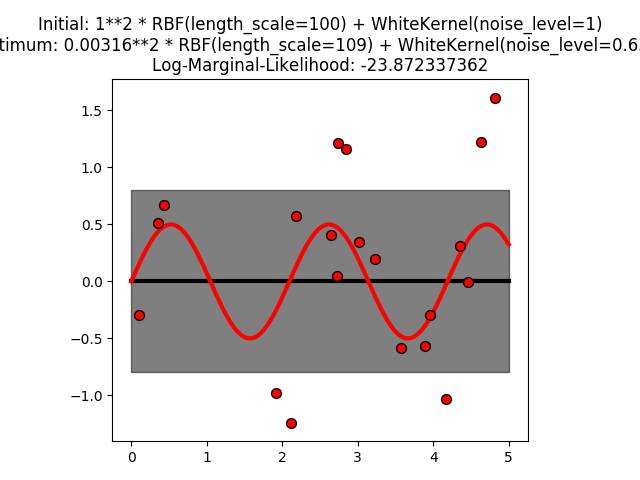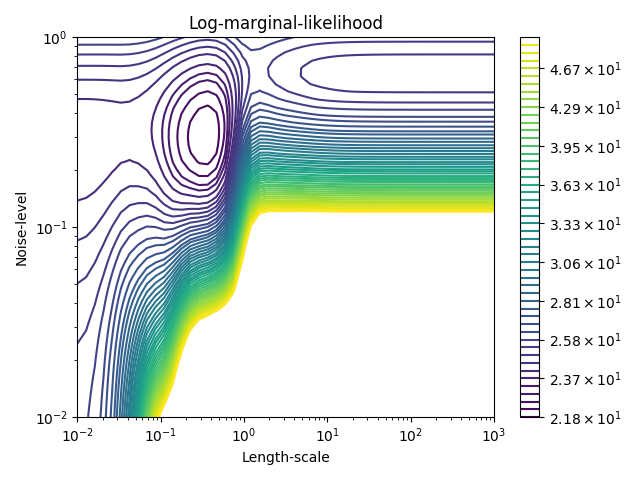### 1.7.2.2. GPR 和内核岭回归（Kernel Ridge Regression）的比较### 1.7.2.3. Mauna Loa CO2 数据中的 GRR

• 一个长期的，顺利的上升趋势是由一个 RBF 内核来解释的。 具有较大长度尺寸的RBF内核将使该分量平滑; 没有强制这种趋势正在上升，这给 GP 带来了这个选择。 具体的长度尺度和振幅是自由的超参数。
• 季节性因素，由定期的 ExpSineSquared 内核解释，固定周期为1年。 该周期分量的长度尺度控制其平滑度是一个自由参数。 为了使准确周期性的衰减，采用带有RBF内核的产品。 该RBF组件的长度尺寸控制衰减时间，并且是另一个自由参数。
• “noise(噪声)” 一词，由一个 RBF 内核贡献组成，它将解释相关的噪声分量，如局部天气现象以及 WhiteKernel 对白噪声的贡献。 相对幅度和RBF的长度尺度是进一步的自由参数。

34.4**2 * RBF(length_scale=41.8)
+ 3.27**2 * RBF(length_scale=180) * ExpSineSquared(length_scale=1.44,
periodicity=1)
+ 0.197**2 * RBF(length_scale=0.138) + WhiteKernel(noise_level=0.0336)## 1.7.3. 高斯过程分类（GPC）

GP先验平均值假定为零。先验的协方差是通过传递 内核(kernel) 对象来指定的。 在通过最大化基于传递的对数边缘似然（LML）的 GaussianProcessRegressor 拟合期间， 优化内核的超参数 optimizer 。由于LML可能具有多个局部最优值， 所以优化器可以通过指定重复启动 n_restarts_optimizer 。 第一次运行始终从内核的初始超参数值开始执行; 从已经从允许值的范围中随机选择超参数值来进行后续运行。 如果初始超参数需要保持固定，None 可以传递作为优化器。

GaussianProcessClassifier 通过执行基于OvR(one-versus-rest)或 OvO(one-versus-one )策略的训练和预测来支持多类分类。 在OvR(one-versus-rest)策略中，每个类都配有一个二进制高斯过程分类器，该类别被训练为将该类与其余类分开。 在 “one_vs_one” 中，对于每对类拟合一个二进制高斯过程分类器，这被训练为分离这两个类。 这些二进制预测因子的预测被组合成多类预测。更多详细信息，请参阅 多类别分类

## 1.7.4. GPC 示例

### 1.7.4.2. GPC 在 XOR 数据集上的举例说明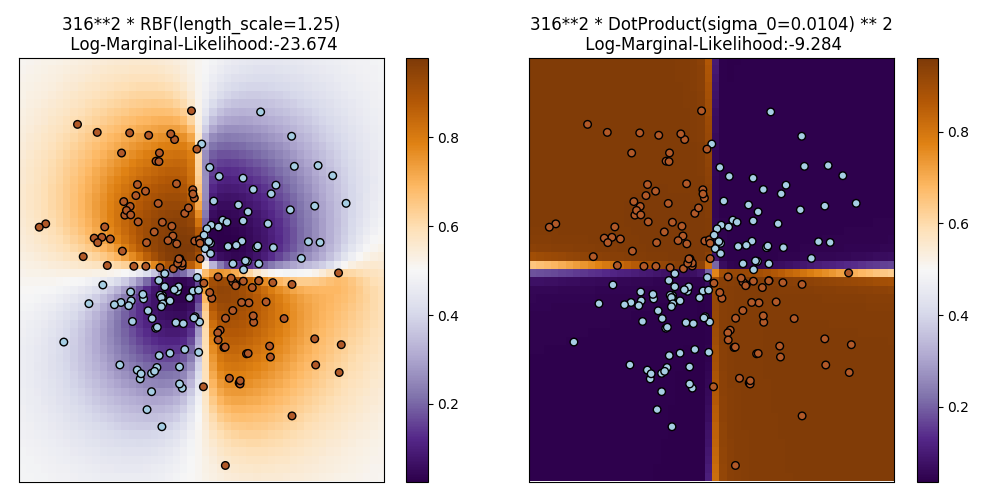### 1.7.4.3. iris 数据集上的高斯过程分类（GPC）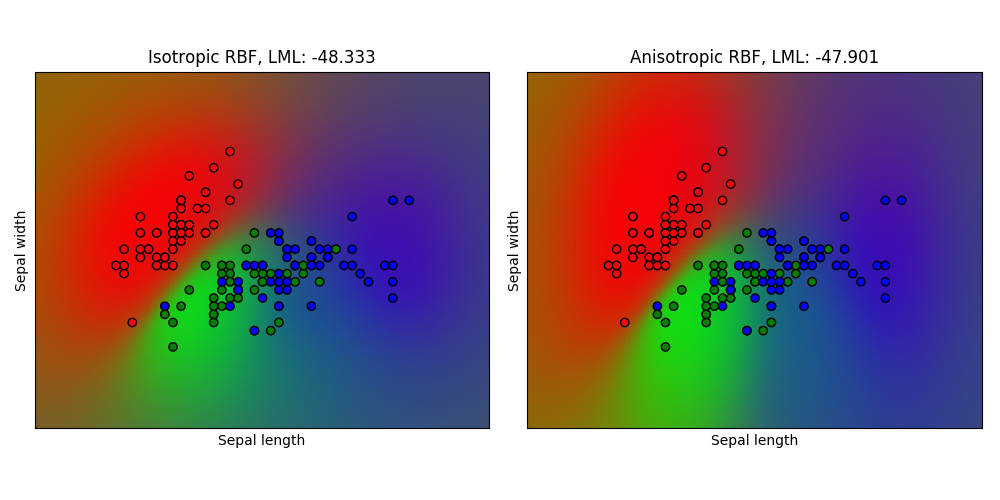## 1.7.5. 高斯过程内核

### 1.7.5.1. 高斯过程内核 API

Kernel 主要是用来计算数据点之间的高斯过程协方差。 为此，内核中 __call__ 方法会被调用。该方法可以用于计算 2d阵列X中所有数据点对的“自动协方差”，或二维阵列X的数据点 与二维阵列Y中的数据点的所有组合的“互协方差”。以下论断对于 所有内核k（除了 WhiteKernel）都是成立的：k(X) == K(X, Y=X)。 如果仅仅是自协方差的对角线元素被使用，那么内核的方法 diag() 将会被调用， 该方法比等价的调用 __call__: np.diag(k(X, X)) == k.diag(X) 具有更高的计算效率。

>>> from sklearn.gaussian_process.kernels import ConstantKernel, RBF
>>> kernel = ConstantKernel(constant_value=1.0, constant_value_bounds=(0.0, 10.0)) * RBF(length_scale=0.5, length_scale_bounds=(0.0, 10.0)) + RBF(length_scale=2.0, length_scale_bounds=(0.0, 10.0))
>>> for hyperparameter in kernel.hyperparameters: print(hyperparameter)
Hyperparameter(name='k1__k1__constant_value', value_type='numeric', bounds=array([[ 0., 10.]]), n_elements=1, fixed=False)
Hyperparameter(name='k1__k2__length_scale', value_type='numeric', bounds=array([[ 0., 10.]]), n_elements=1, fixed=False)
Hyperparameter(name='k2__length_scale', value_type='numeric', bounds=array([[ 0., 10.]]), n_elements=1, fixed=False)
>>> params = kernel.get_params()
>>> for key in sorted(params): print("%s : %s" % (key, params[key]))
k1 : 1**2 * RBF(length_scale=0.5)
k1__k1 : 1**2
k1__k1__constant_value : 1.0
k1__k1__constant_value_bounds : (0.0, 10.0)
k1__k2 : RBF(length_scale=0.5)
k1__k2__length_scale : 0.5
k1__k2__length_scale_bounds : (0.0, 10.0)
k2 : RBF(length_scale=2)
k2__length_scale : 2.0
k2__length_scale_bounds : (0.0, 10.0)
>>> print(kernel.theta)  # Note: log-transformed
[ 0.         -0.69314718  0.69314718]
>>> print(kernel.bounds)  # Note: log-transformed
[[      -inf 2.30258509]
[      -inf 2.30258509]
[      -inf 2.30258509]]


### 1.7.5.2. 基础内核

ConstantKernel 内核类可以被用作 Product 内核类的一部分， 在它可以对其他因子（内核）进行度量的场景下或者作为更改高斯过程均值的Sum 类的一部分。这取决于参数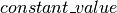的设置。该方法定义为：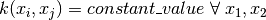WhiteKernel 内核类的主要应用实例在于当解释信号的噪声部分时 可以作为内核集合的一部分。通过调节参数， 该类可以用来估计噪声级别。具体如下所示：### 1.7.5.4. 径向基函数内核

RBF 内核是一个固定内核，它也被称为“平方指数”内核。它通过定长的参数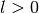来对内核进行参数化。该参数既可以是标量（内核的各向同性变体）或者与输入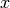（内核的各向异性变体） 具有相同数量的维度的向量。该内核可以被定义为：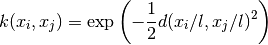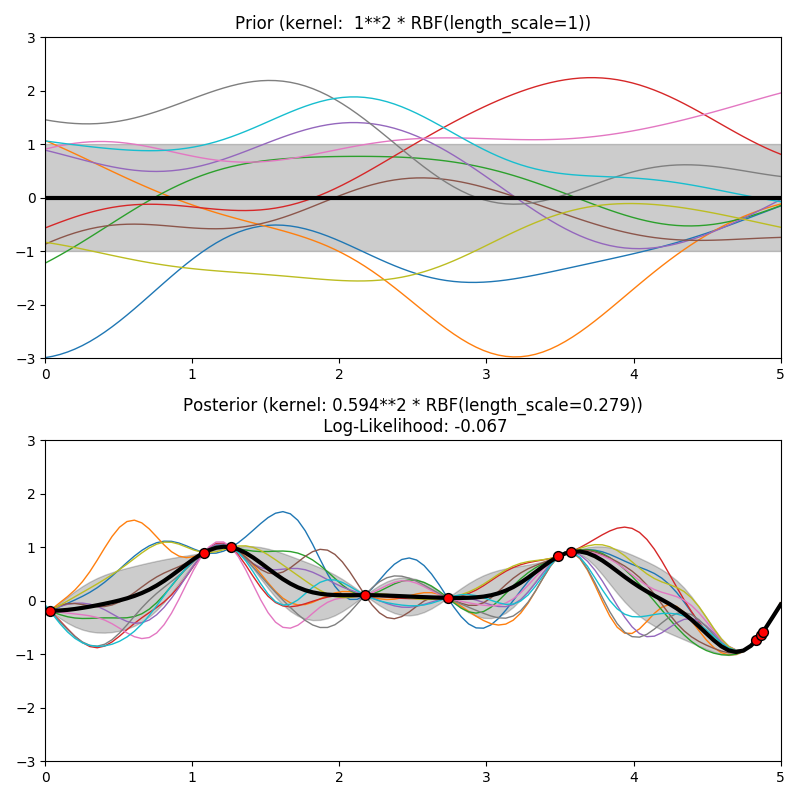### 1.7.5.5. Matérn 内核

Matern 内核是一个固定内核，是 RBF 内核的泛化。它有一个额外的参数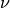， 该参数控制结果函数的平滑程度。它由定长参数来实现参数化。该参数既可以是标量 （内核的各向同性变体）或者与输入（内核的各向异性变体）具有相同数量的维度的向量。 该内核可以被定义为：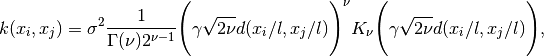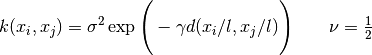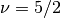: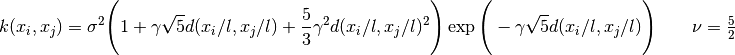### 1.7.5.6. 有理二次内核

RationalQuadratic 内核可以被看做不同特征尺度下的 RBF 内核的规模混合（一个无穷和） 它通过长度尺度参数和比例混合参数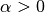进行参数化。 此时仅支持标量的各向同性变量。内核公式如下：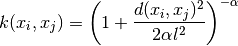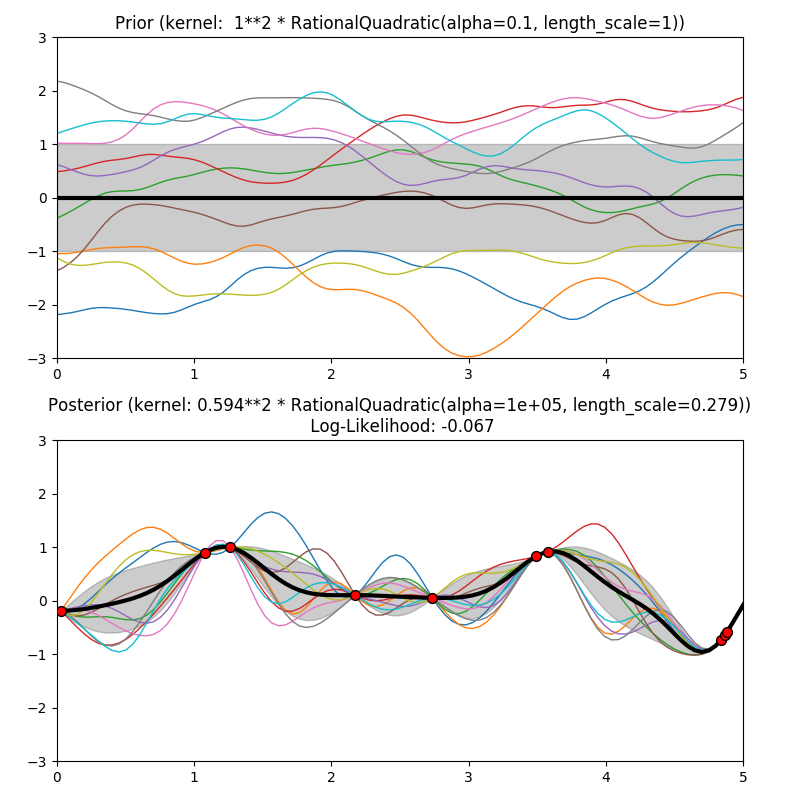### 1.7.5.7. 正弦平方内核

ExpSineSquared 内核可以对周期性函数进行建模。它由定长参数以及周期参数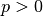来实现参数化。此时仅支持标量的各向同性变量。内核公式如下：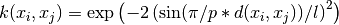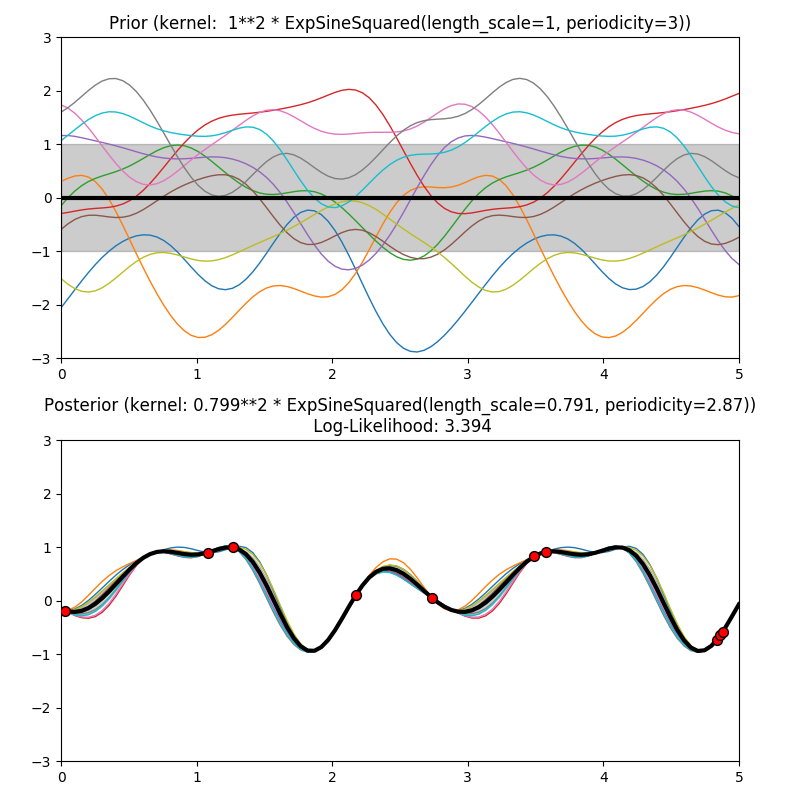### 1.7.5.8. 点乘内核

DotProduct 内核是非固定内核，它可以通过在线性回归的的相关系数上加上 服从于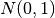的先验以及在线性回归的偏置上加上服从于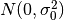的先验来获得。 该 DotProduct 内核对于原点坐标的旋转是不变的，因此不是转换。它通过设置参数来进行参数化。 当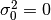时，该内核叫做同质线性内核；否则该内核是非同质的。内核公式如下：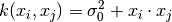DotProduct 内核通常和指数分布相结合。实例如下图所示：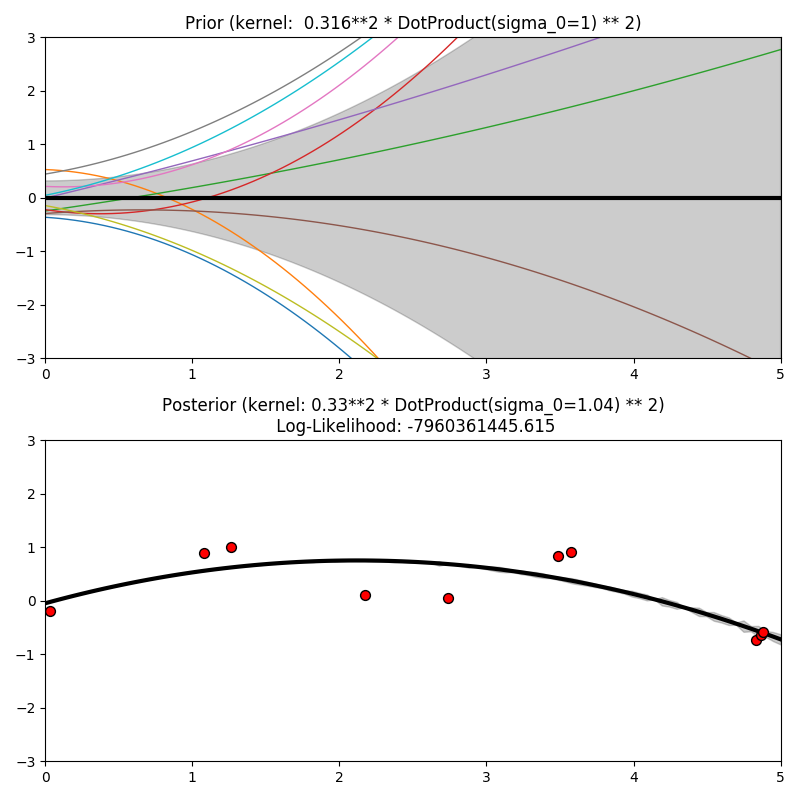### 1.7.5.9. 参考文献

[RW2006] Carl Eduard Rasmussen and Christopher K.I. Williams, “Gaussian Processes for Machine Learning”, MIT Press 2006, Link to an official complete PDF version of the book here .